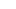# Tips for easy and fast calculations for CAT 2013

Photo Credit: thethreesisters

When you are competing against thousands or rather lakhs of people for a few seats, every minute matters. These days, probably what you did in your class 10th, 12th and graduation matters more than most things – but hey, you cannot do anything about that until someone invents a time machine. Although it can be debated whether it is fair / unfair / valid, etc. but I would like to reiterate – you cannot do anything about that. So, let us concentrate on something that you can do. You can work hard. You can do well in CAT and other exams. Here are a few tips and tricks which you can use to calculate faster in an exam.

Calculating Squares

Type 1: Numbers ending in 5

If we remove the last digit ‘5’ from our number and are left with x, then the square will be [Product of x and x+1]25.

115^2

First part = 11 * 12 = 132

Last part = 25

395^2

First part = 39 * 40 = 1560

Last part = 25

Type 2: Numbers close to 50 [41,59]

Let the distance of the number from 50 be ‘d’. Then the square will be [25+d][d^2].

53^2

Distance from 50 = 3

First part = 25 + 3 = 28

Last part = 3^2 = 9 = 09

44^2

Distance from 50 = – 6

First part = 25 – 6 = 19

Last part = (-6)^2 = 36 = 36

Type 3: Numbers close to 100 [91,109]

Let the distance of the number ‘n’ from 100 be ‘d’. Then the square will be [n+d][d^2].

107^2

Distance from 100 = 7

First part = 107 + 7 = 114

Last part = 7^2 = 49

98^2

Distance from 100 = – 2

First part = 98 – 2 = 96

Last part = (-2)^2 = 4 = 04

Type 4: Numbers ending in 1,4,6,9

Calculate the square of the nearest multiple of 5. Add or subtract (Num + Multiple of 5) from it. If the multiple of 5 is bigger than the given number, then you subtract – otherwise add.

71^2

Nearest multiple of 5 = 70

Square of the nearest multiple of 5 = 70^2 = 4900

Number + Multiple of 5 = 70 + 71 = 141

Answer = 4900 + 141 = 5041

134^2

Nearest multiple of 5 = 135

Square of nearest multiple of 5 = 135^2 = [13*14] = 18225

Number + Multiple of 5 = 134 + 135 = 269

Answer = 18225 – 269 = 17956

Now some of you might be thinking, “Where am I going to use this? This will come in handy in very few situations.” Well, I agree with you. Prima facie, it does look like something which might not come in handy on the first day or even the first week. But if you spend 10 minutes practicing the above tips, I can guarantee that you will save lot more time during your preparation. And if you can save some time during the exam, well that is a big fat bonus.

Having said that, given below is something that you can start using from today.

Multiplying Two Digit Numbers

In your normal way of multiplication, when you multiply 32 with 41, this is what you normally do:For calculating faster, this is what you should do:The idea here is instead of two steps, you should calculate the answer in just one step. Consider your answer as a combination of three parts

Last digit = Product of the last digits (may generate a carry, does not in this case) = 2 * 1 = 2

Second last digit = Cross product of the digits (may generate a carry, does in this case) = 3 * 1 + 2 * 4 = 3 + 8 = 11 = 1 written down and 1 carry

First part = Product of first digits (might include previous carry, does include in this case) = 4 * 3 + 1 = 1 3and